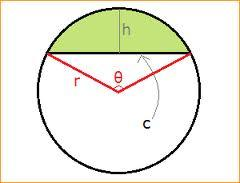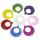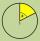# Arc and segment

Calculate the length of circular arc l, area of the circular arc S1 and area of circular segment S2. Radius of the circle is 11 and corresponding angle is
$\frac{2}{12}\pi$
.

l =  5.8
S1 =  31.7
S2 =  1.4

### Step-by-step explanation:

$l=\frac{2}{12}\pi \cdot 11=5.8$
${S}_{1}=\frac{2}{2\cdot 12}\pi \cdot 1{1}^{2}=31.7$
${S}_{2}=\frac{1}{2}\cdot 1{1}^{2}\left(\frac{2}{12}\pi -\mathrm{sin}\frac{2}{12}\pi \right)=1.4$Did you find an error or inaccuracy? Feel free to write us. Thank you!Tips to related online calculators
Most natural application of trigonometry and trigonometric functions is a calculation of the triangles. Common and less common calculations of different types of triangles offers our triangle calculator. Word trigonometry comes from Greek and literally means triangle calculation.

#### You need to know the following knowledge to solve this word math problem:

We encourage you to watch this tutorial video on this math problem:

## Related math problems and questions:

• Circle arcCircle segment has a circumference of 135.26 dm and 2096.58 dm2 area. Calculate the radius of the circle and size of the central angle.
• Arc-sectorarc length = 17 cm area of sector = 55 cm2 arc angle = ? the radius of the sector = ?
• Circle sectorThe circular sector with a central angle 160° has an area 452 cm2. Calculate its radius r.
• Circular segmentCalculate the area S of the circular segment and the length of the circular arc l. The height of the circular segment is 2 cm and the angle α = 60°. Help formula: S = 1/2 r2. (Β-sinβ)
• Circle and angleWhat is the length of the arc of a circle with radius r = 207 mm with cental angle 5.33 rad?
• SectorThe perimeter of a circular sector with an angle 1.8 rad is 64 cm. Determine the radius of the circle from which the sector comes.
• ArcCircle arc corresponding to angle is 32° is 28 dm long. What is the length of the entire circle?Calculate the content of a regular 15-sides polygon inscribed in a circle with radius r = 4. Express the result to two decimal places.
• PentagonCalculate the length of side, circumference and area of a regular pentagon, which is inscribed in a circle with radius r = 6 cm.
• ArcWhat area of a circle occupied the flowers planted in the arc of a circle with radius 3 m with central angle 45°?
• Circle arcCalculate the circular arc area in m2 where the diameter is 263 dm, and a central angle is 40°. Result round to three decimal places.
• DiscThe circumference of the disk is 78.5 cm. What is the circumference of the circular arc of 32° on the disc?
• Circle sectionEquilateral triangle with side 33 is inscribed circle section whose center is in one of the vertices of the triangle and the arc touches the opposite side. Calculate: a) the length of the arc b) the ratio betewwn the circumference to the circle sector and
• Quarter of a circleCalculate the circumference of a quarter circle if its content is S = 314 cm2.Calculate the radius of the quadrant, which area is equal to area of circle with radius r = 15 cm.The wire that is hooked around the perimeter of the quarter-circular arc has length 3π+12. Determine the radius of the circle arc.How much fuel is in the horizontal cylindrical segment tank with a length of 10m, the width of level 1 meter, and the level is 0.2 meters below the tank's upper side?